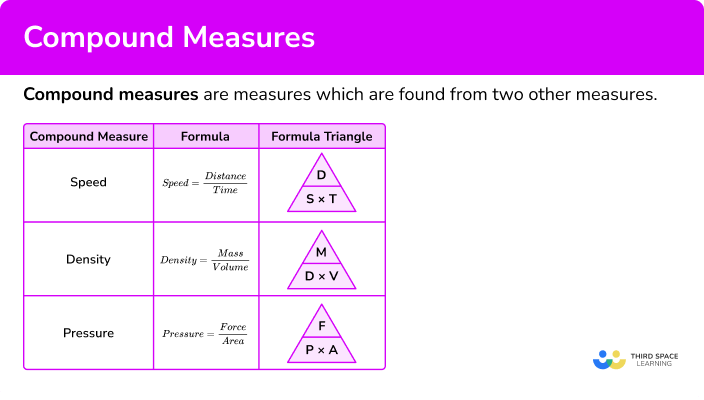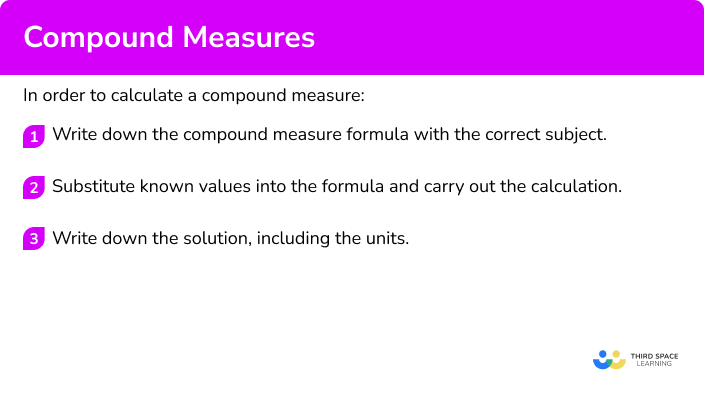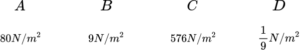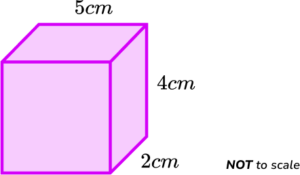GCSE Maths Ratio and Proportion

Compound Measures

# Compound Measures

Here we will learn about compound measures including speed, density and pressure and look at problem solving using compound measures.

There are also compound measures worksheets based on Edexcel, AQA and OCR exam questions, along with further guidance on where to go next if you’re still stuck.

## What are compound measures?

Compound measures are measures which are found from two other measures.

The most common compound measures studied in GCSE maths are speed, density, and pressure.

To calculate the value of a compound measure, we need to know the value and the units of the other two measures

### What are compound measures?### ● Speed

Speed is a compound measure made from dividing a unit of distance by a unit of time. Speed is therefore a measure of how much an object has travelled over a certain time period.

Common units for speed include metres per second (m/s) and kilometres per hour (km/h). Miles per hour (mph) is associated with the speed of a vehicle. At GCSE we tend to use metric units rather than imperial units.

Step-by-step guide: Speed, distance, time

Speed formula

To calculate the speed of an object, we use the speed formula,

\text{Speed}=\frac{\text{distance}}{\text{time}} .

This can be written as a formula triangle.

Step-by-step guide: Formula for speed

### ● Density

Density is a compound measure made from dividing a unit of mass by a unit of volume. Density is a measure of how much matter there is in a certain volume.

Common units for density include, grams per cubic centimetre (g/cm^{3}) and kilograms per cubic metre (kg/m^{3}).

Step-by-step guide: Mass, density, volume

Density formula

To calculate the density of an object, we use the density formula,

\text{Density}=\frac{\text{mass}}{\text{volume}} .

This can be written as a formula triangle.

Step-by-step guide: Formula for density

### ● Pressure

Pressure is a compound measure made from dividing a unit of force by a unit of area. Pressure is therefore defined as the force per unit area. Different units of pressure include Pascals (Pa) where one Pascal is equivalent to 1 \ N/m^{2}.

Step-by-step guide: Pressure, force, area

Pressure formula

To calculate the pressure an object makes with a surface, we use the pressure formula,

\text{Pressure}=\frac{\text{force}}{\text{area}} .

This can be written as a formula triangle.

Step-by-step guide: Pressure formula

There are other compound measures such as rates of pay (for example, £ per hour). We can also use compound measures when calculating the best buy (for example, grams per pence).

### ● Compound units

Compound units are the units of measurement used with compound measures.

For example, the compound units for speed include metres per second (m/s), kilometres per hours (km/h) and miles per hour (mph).

The compound units for density include grams per cubic centimetre (g/cm^{3}) and kilogram per cubic metre (kg/m^{3}).

## How to calculate a compound measure

In order to calculate a compound measure:

1. Write down the compound measure formula with the correct subject.
2. Substitute known values into the formula and carry out the calculation.
3. Write down the solution, including the units.

### Explain how to calculate a compound measure## Compound measures examples

### Example 1: speed

A car travels 100 kilometres in 2 hours. Calculate the average speed of the car.

1. Write down the compound measure formula with the correct subject.

To calculate the speed, we divide the distance by the time.

S=\frac{D}{T}

2Substitute known values into the formula and carry out the calculation.

Substituting the values D=100 and T=2, we have

S=\frac{D}{T}=\frac{100}{2}=50.

3Write down the solution, including the units.

The average speed of the car is 50 \ km/h.

### Example 2: speed

A train travels at a speed of 120 \ km/h for 1\frac{1}{2} hours. Calculate the distance the train has travelled in this time.

To calculate the distance, we need to multiply the speed by the time.

D=S\times{T}

As S=120 and T=1\frac{1}{2}=1.5, substituting these into the formula, we have

D=S\times{T}=120\times{1.5}=180.

The train travelled 180 \ km.

### Example 3: density

A platinum bar has a volume of 40 \ cm^{3} and mass of 840 \ g. Calculate the density of the platinum bar.

To calculate the density, we need to divide the mass of the object by its volume.

D=\frac{M}{V}

As M=840 and V=40, we have

D=\frac{M}{V}=\frac{840}{40}=21.

The density of the platinum bar is 21 \ g/{cm}^{3}.

### Example 4: density

A steel block has a mass of 1.17 \ kg. The density of steel is 7.8 \ g/cm^{3}. Calculate the volume of the steel block.

To calculate the volume, we divide the mass of the object by the density of the substance.

V=\frac{M}{D}

Here we have been given the mass in kilograms, but the density is in g/cm^{3} and so we need to convert the mass to grams first.

1.17 \ kg=1170 \ g.

Now that we have the mass in grams, we can calculate the volume of the steel block.

V=\frac{M}{D}=\frac{1170}{7.8}=150.

The volume of the steel block is 150 \ {cm}^{3}.

### Example 5: pressure

A force of 820 \ N is exerted on an area of 40 \ m^{2}. Calculate the pressure acting on the area.

To calculate the pressure we divide the force by the area.

P=\frac{F}{A}

As F=820 and A=40, we have

P=\frac{F}{A}=\frac{820}{40}=20.5.

The pressure is 20.5 \ N/m^2 or 20.5 \ Pa.

### Example 6: pressure

A force of 860 \ N exerts a pressure of 47 \ N/m^{2}. Calculate the area the force is being applied to. Write your answer correct to 3 significant figures.

To calculate the area we need to divide the force by the pressure.

A=\frac{F}{P}

As F=860 and P=47, substituting these into the formula, we have

A=\frac{F}{P}=\frac{860}{47}=18.2978723404…

The area the force is applied to is 18.3 \ m^2 \ (3sf).

### Common misconceptions

Check that you give the answer in the right form. You may have an answer which needs to be rounded to a specific number of significant figures or decimal places.

• Average speed

Sometimes a journey may be in more than one part. You may be asked to find the average speed. To do this we would divide the total distance travelled by the total time taken.

\text{Average speed}=\frac{\text{total distance}}{\text{total time}}

### Practice compound measures questions

1. A bus travels for 120 \ km in 3 hours. Calculate the average speed of the bus.

360 \ km/h0.025 \ km/h40 \ km/h30 \ km/h\text{Speed}=\frac{\text{distance}}{\text{time}}=\frac{120}{3}=40 \ km/h

2. A person cycles at a speed of 10 \ km/h for 25 \ km. Calculate the time taken for the cyclist to complete the journey.

250 hours3.5 hours2.5 hours35 hours\text{Time}=\frac{\text{distance}}{\text{speed}}=\frac{25}{10}=2.5 hours

3. Calculate the density of an object with a mass of 350 \ g and a volume of 25 \ cm^{3}.

14\ g/cm^3325\ g/cm^3375\ g/cm^38750\ g/cm^3\text{Density}=\frac{\text{mass}}{\text{volume}}=\frac{350}{25}=14 \ g/cm^3

4. The density of a substance is 9 \ g/cm^{3} and its volume is 280 \ cm^{3}. Calculate the mass of the object in kg.

2.52 \ kg31.1 \ kg32.1 \ kg289 \ kg\text{Mass}=\text{density}\times \text{volume}=9\times 280=2520\ g

Converting grams to kilograms, we have

2520\ g= 2.52\ kg.

5. A force of 70 \ N acts on an area of 14 \ m^{2}. Calculate the pressure.

0.2 \ N/m^25 \ N/m^256 \ N/m^284 \ N/m^2\text{Pressure}=\frac{\text{force}}{\text{area}}=\frac{70}{14}=5 \ N/m^2

6. A pressure of 53 \ N/m^{2} is exerted on an area of 4 \ m^{2}. Calculate the force the object applies to the area.

57 \ N13.25 \ N848 \ N212 \ N\text{Force}=\text{pressure}\times \text{area}=53\times 4=212 \ N

### Compound measures GCSE questions

\text{Density}=\frac{\text{mass}}{\text{volume}} \hspace{3cm} \text{Pressure}=\frac{\text{force}}{\text{area}}

1. Work out the pressure when the force is 72 \ N and the area is 8 \ m^{2}.(1 mark)

B – 9 \ N/m^2

(1)

2. (a) Calculate the volume of the cuboid below.(b) The cuboid is made from copper.

The density of copper is 8.6 \ g/cm^{3}.

Calculate the mass of the cuboid.

(4 marks)

(a)

5\times 4\times 2

(1)

40 \ cm^3

(1)

(b)

8.6\times 40

(1)

344 \ g

(1)

3. (a) A bee flies from its hive to a flower.

It flies at a constant speed of 9 metres per second for 12 seconds.

Calculate the distance from the hive to the flower.

(b) The bee flies back to the hive.

It takes 15 seconds to return.

Calculate the speed of the bees return flight.

(4 marks)

(a)

9 \times 12

(1)

108 \ m

(1)

(b)

108\div 15

(1)

7.2 \ m/s

(1)

4. Population density is calculated by using this formula,

\text{Population density}=\frac{\text{population}}{\text{area}} .

The population of Belgium is 11 \ 482 \ 178 people and has an area of 30 \ 528 \ km^{2}.

Calculate the population density of Belgium.

(3 marks)

11 \ 482 \ 178 \div 30 \ 528=376.11956236897…

(1)

374 \ (3sf)

(1)

people/km^2

(1)

## Learning checklist

You have now learned how to:

• Use compound units such as speed, unit pricing and density to solve problems

## Still stuck?

Prepare your KS4 students for maths GCSEs success with Third Space Learning. Weekly online one to one GCSE maths revision lessons delivered by expert maths tutors.

Find out more about our GCSE maths tuition programme.